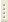06.02.2023Introduction to Semiconductor Physics

Prof. V.D.Kulakovskii

The course is devoted to the fundamentals of semiconductor physics. The principal divisions as well as current problems (polaritons in bulk semiconductors and semiconductor quasi-two-dimensional nanostructures) of semiconductor physics are considered.

Curriculum

1. Introduction. Kinetic phenomena in semiconductors: electrical conduction, quantum Hall effect, magnetic zero and temperature gradient effects. Mean free time.
2. Elementary theory of galvanomagnetic effects (magnetic field electrical conductivity tensor), Hall angle and Hall constant, magnetoresistance), mixed conductivity, experimental measure measurements of conductivity and quantum Hall effect.
3. Chemical bonds in semiconductors. Crystal lattices, electron configuration of atoms. Types of chemical bond: ion bond, homopolar bond, Van der Waals chemical bond, mixed bond crystal, noncrystalline semiconductors.
4. Semiconductor properties and chemical bond. Forbidden band, impurity levels, crystal vacancies.
5. Elements of band theory of semiconductors (perfect lattice). Basic assumptions of band theory. Wave electron function in periodic field, Brillouin zones, energy bands.
6. Strongly coupled electron method.
7. Electron and hole dispersion law. Effective mass. Examples of semiconductor band structure.
8. Elements of semiconductor band theory (semiconductors in external fields, imperfect crystals). Average values of electron acceleration and velocity, electrons and holes in magnetic field (classical theory), diamagnetic resonance.
9. Effective mass method. Electron and hole energy spectra in constant electric field (quantum theory), shallow impurity levels.
10.  Electron and hole statistics in semiconductors. Band distribution of quantum states, Fermi-Dirac distribution, effective state density in bands, carrier concentration in degenerate and nondegenerate semiconductors, electron and hole concentration on local levels.
11.  Gibbs distribution. Determination of Fermi level position in intrinsic semiconductor and doped semiconductors.
12.  Contact phenomena. Potential barriers, current density, Einstein relation, body equilibrium condition, thermionic work function, contact potential difference.
13. Distribution of electron and potential concentration in bulk charge layer, screening length, enriched and depleted layer. Metal-semiconductor rectification.
14.  Non-equilibrium electrons and holes in semiconductors. Non-equilibrium electron and hole lifetime, continuity equation, photoconductivity, Fermi quasilevels.
15.  Problems of band theory justification. Adiabatic approximation, small oscillation approximation, self-consistent field method.
16. Polaritons, excitons, exciton molecules, exciton ionization, electron-hole plasma, electron-hole liquid.
17. Polaritons in bulk semiconductors and semiconductor quasi-two-dimensional nanostructures.
18.  Polaritons in semiconductor microresonators.